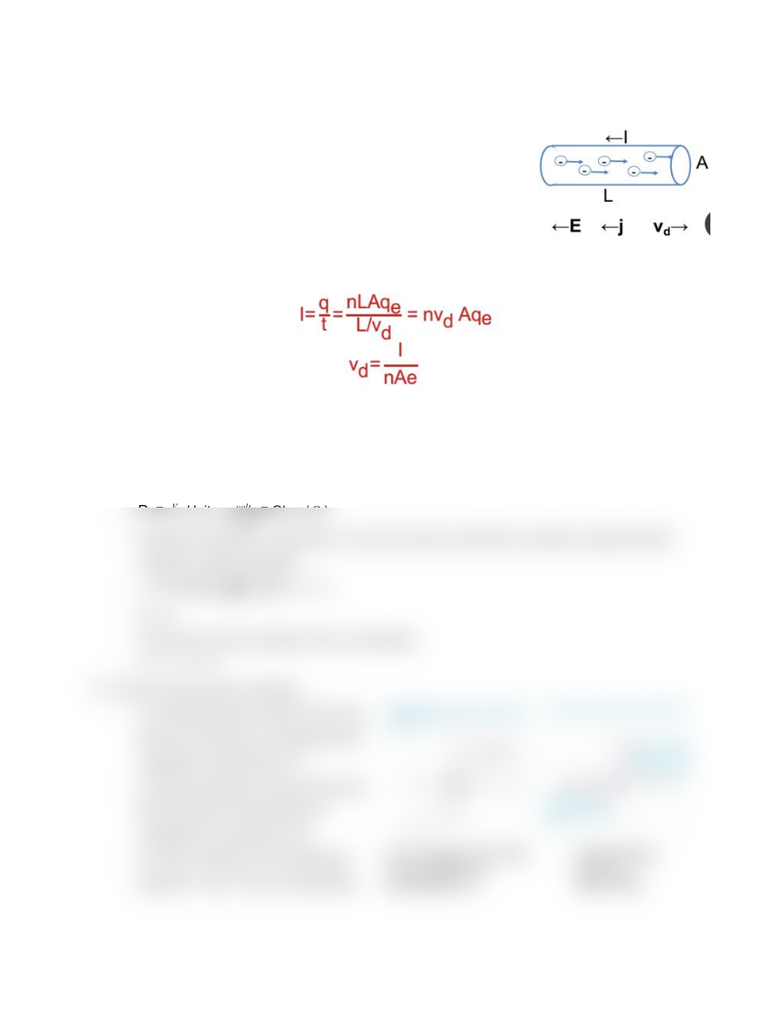Get 2 days of premium access
Class Notes (1,000,000)
US (430,000)
UC-Irvine (10,000)
PHYSICS (500)
Lecture 12

# PHYSICS 7D Lecture Notes - Lecture 12: Ampere, Joule, VoltmeterPremium

Department
Physics
Course Code
PHYSICS 7D
Professor
William Molzon
Lecture
12

This preview shows half of the first page. to view the full 2 pages of the document.Lecture 12: Currents and Resistance
I. Drift Velocity
- In a conductor of length L and area A, the number of
conduction electrons = nV= nLA, where n is the
number of conductor electrons per unit volume.
- An electron takes a time t= L/vd to pass through this
length of conductor: in time L/vd, all free charge in
length L exits through the downstream end, so
II. Resistance and Resistivity
- Ohm’s Law: For some materials, the current through a piece of material is directly
proportional to the voltage difference between the two points. For these materials, the
constant R that relates the voltage and the current is called the resistance.
- R Units: Ohm ( )
I
V volt
ampere Ω
- Resistance depends on geometry. If we don’t want to deal with a particular object but with
materials- define resistivity
- Units: = m = * mj
E V /m
A/m2A
VΩ
- E = pj
- The inverse of the resistivity is the conductivity:
- j= Eσ1
σ
III. Ohmic and non-ohmic materials
- A conducting device obeys Ohm’s law
when the resistance is independent of
magnitude and polarity of V.
- For these materials, Ohm's law means
that resistivity is independent of
magnitude and direction of E.
- For other materials, the relationship
between V and I can be complicated.
###### You're Reading a Preview

Unlock to view full version

Subscribers Only